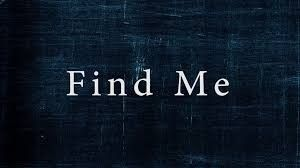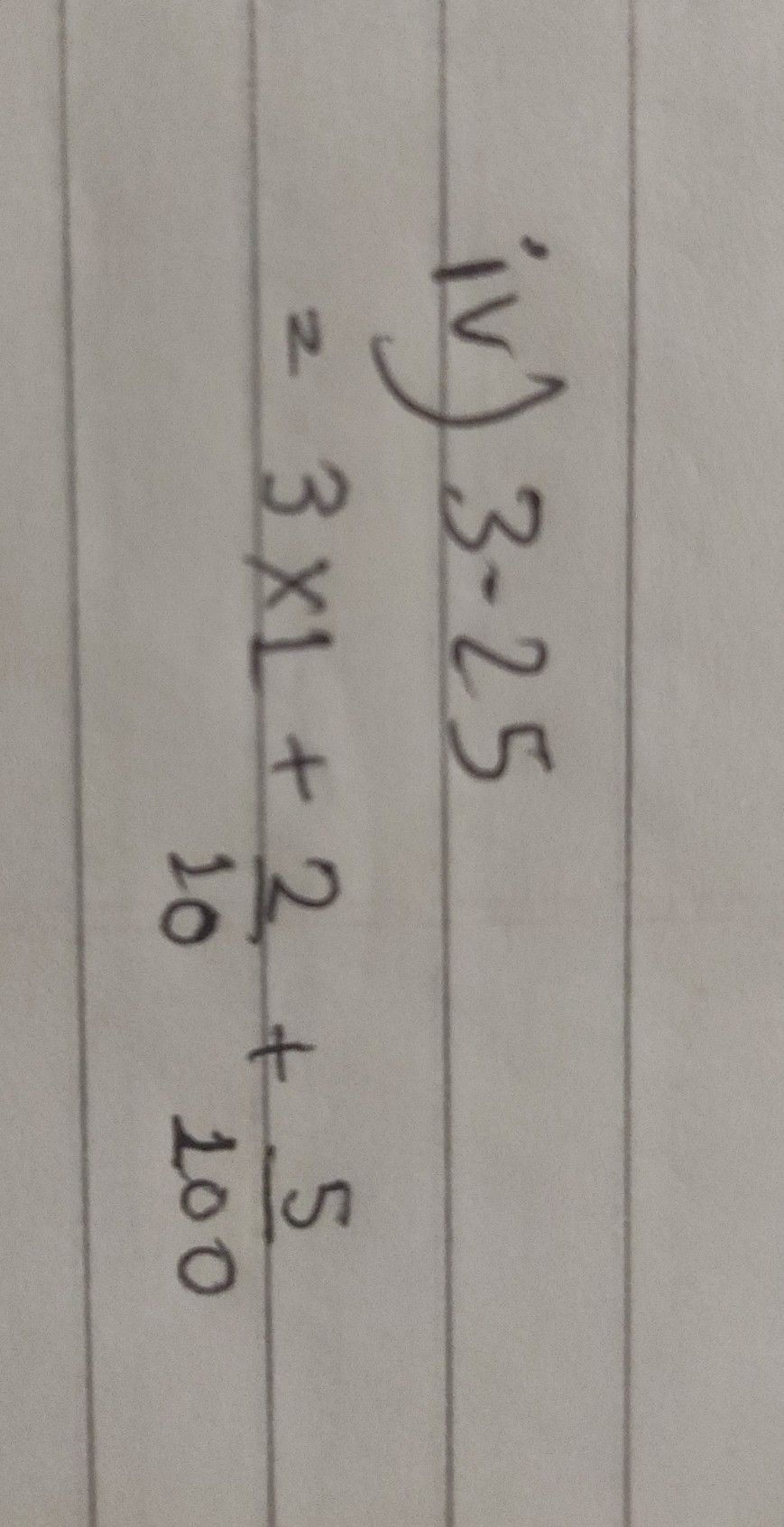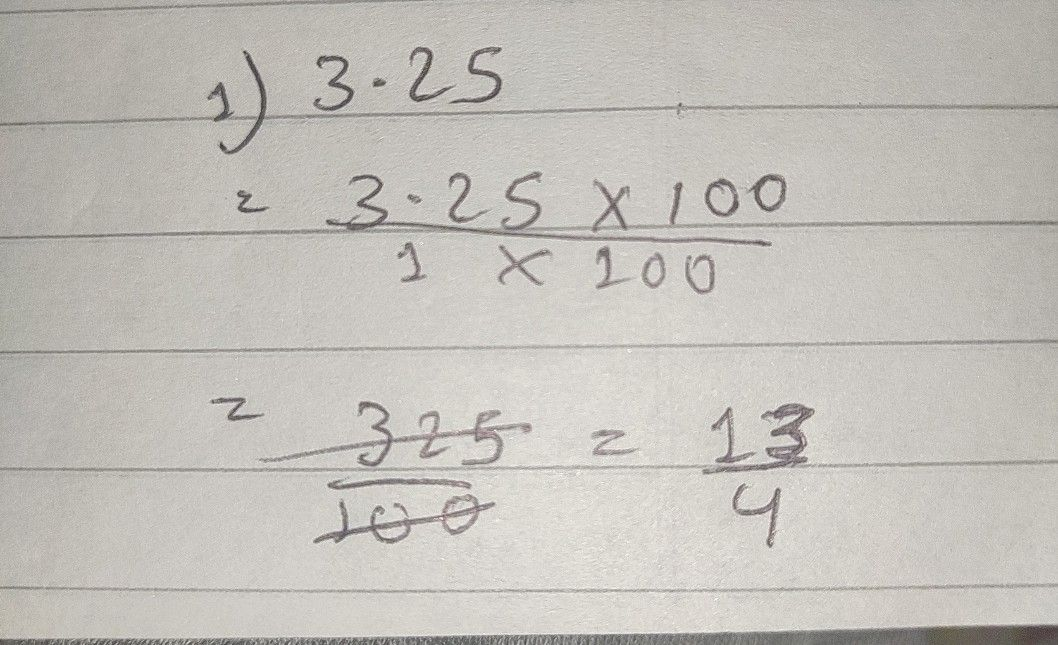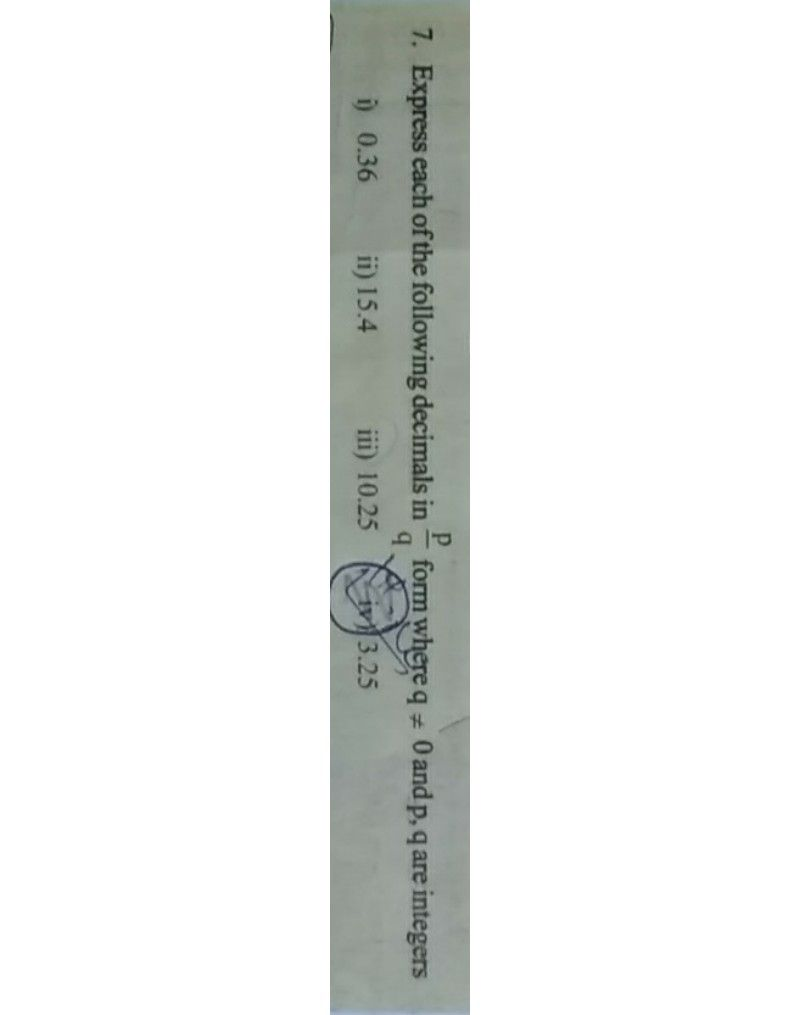Symbol
Problem$1$ $2$ $77$ $3.25$
Other
SolutionQanda teacher - FINDERStudent
sir but the back answer in our book is 13/4Qanda teacher - FINDER
what are we exactly supposed to do in this question
send me the complete photo of the questionStudent
ok sirQanda teacher - FINDER